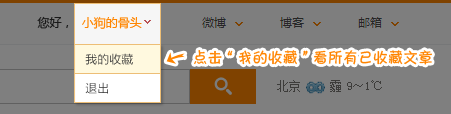# [500彩票网]神采飞扬双色球075期：龙头首选1路

2016年06月29日11:15    新浪彩票 收藏本文

[500彩票网]神采飞扬双色球2016075期： 胆码01、03

龙头推荐：

01 03 09 首选1路，看淡2路。

凤尾推荐：

29 31 32 首选1路，看淡0路。

胆码推荐：

金胆：01

银胆：03

铜胆：32

胆组：01+03+09+32

双胆：01+04、03+09、13+17、23+29、29+31

冷号推荐： 01+04

重号推荐：11+20

尾号推荐：1+2+3+9

连号推荐：03+04、07+08、18+19、20+21、31+32

蓝色狂想曲：

［八码围蓝］：03+06+07+08+09+10+13+16

［六码围蓝］：06+07+08+09+10+16

［四码定蓝］：06+08+09+10

［三码定蓝］：06+08+09

［二码定蓝］：06+09

［一码擒蓝］：09

号码组合：

非常6+5：

红球：01+03+09+13+17+32

蓝球： 06+08+07+09+10

8码小复式：

红球：01+03+09+13+17+29+31+32

蓝球：06+08+09

12码大复式：

红球： 01+03+04+09+13+17+20+21+23+29+31+32

蓝球： 09

20码选号大底：

01+03+04+07+08+09+11+13+14+17+18+19+20+21+23+26+28+29+31+32